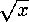<b>double sqrt(double x)</b>

Document: The C Standard LibraryConclusionMathematical Functions: math.hdouble pow(double xdouble y)

## double sqrt(double x)

The return value is the square root of x, i.e.. x must be greater than or equal to zero.

McMullin@eeng.dcu.ie
Fri Mar 29 14:35:38 GMT 1996